[中文] Search Internet Search HelloDBA
Oracle Technology Site. email: fuyuncat@gmail.com  MSN: fuyuncat@hotmail.com# CBO Statistics data gathering and internal arithmetic analysis -- Frequency or Height Balanaced?

[中文]

Author:  fuyuncat

Source:  www.HelloDBA.com

Date:  2010-01-11 06:13:34

Share to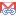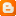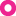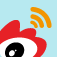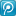## 2. How to choose histogram, Frequency or Height Balanced?

Frequency and Height Balanced are two methods to create histogram correspond to different data values distribution. Their grouping keys are different, endpoint number meaning are different and other dependent stats data such as density are different.

When choose frenquency or height balanced histogram, oracle relyes on some data, such as Number of Distinct Values(NDV), sample size, density and the finally buckets number. However, to the finally buckets number, it requires to create the histogram. So, oracle will first try to create histogram using one kind of method dependending on some simple conditions, then check it fits its requirements or not. If not matched, oracle will convert the histogram to the other kind of histogram or create new histogram using the other kind of method. We can use below pseudocode descript the process.

SQL代码
1. Estimate NumberofDistinctValues (NDV)
2. Get the MaxNumberofBucket(MNB) correspond to Bucket SIZE setting
3.
4. if NDV < 0.9*2000 then
5.   Try to create frequency histogram
6.   if frequency histogram is ok then
8.
9. if Not created frequency histogram or the created frequency histogram is not ok then
10.   Try to create height balanced histogram
11.   if height balanced histogram is ok then
12.     if not tried frequency histogram and sample NDV less than MNB - 1 then
13.        Try to create frequency histogram
14.        if frequency histogram is ok then
16.        else
18.
19. If no histogram be adopted then
20.   adjust sample PCT and repeat the process again

Note: we will discuss the Sample PCT in another paper.

Estimated NDV depends on sample NDV or Numberof Notnull Values(NNV), and the Maximum Number of Bucket(MNB) is calculated from bucket size setting. I use perl script demo the calculation of these 2 values:

SQL代码
1. HELLODBA.COM>host perl get_MNB.pl
2. Sample percent is 30%
3. Sample number of Not Null Values is 100, calculated number of Not Null Values is 333.333333333333
4. Sample Number of Distinct Values is 50, Estimated Number of Distinct Values is 50
5. SIZE setting is , calculated Maximum Number of Bucket is 75

Then, let's further investigate the histogram creating process and see which one finally be adopted.

### Frequency Histogram

Oracle first construct a query to get the Frequency Histogram data. We can find such query by tracing.

SQL代码
1. HELLODBA.COM>set serveroutput on
2. HELLODBA.COM>begin
3.   2   dbms_output.enable(1000000);
4.   3   dbms_stats.set_param('TRACE',2049);
5.   4   dbms_stats.gather_table_stats('DEMO','T_TEST7',NULL,0,FALSE,'FOR COLUMNS OBJECT_TYPE');
6.   5  end;
7.   6  /
8. select /*+ no_parallel(t) no_parallel_index(t) dbms_stats cursor_sharing_exact use_weak_name_resl dynamic_sampling(0) no_monitoring */ count(*)
9. ,count("OBJECT_NAME"),sum(sys_op_opnsize("OBJECT_NAME"))
10. ,count("SUBOBJECT_NAME"),sum(sys_op_opnsize("SUBOBJECT_NAME"))
11. ,count("OBJECT_ID"),sum(sys_op_opnsize("OBJECT_ID"))
12. ,count("DATA_OBJECT_ID"),sum(sys_op_opnsize("DATA_OBJECT_ID"))
13. ,count("OBJECT_TYPE"),sum(sys_op_opnsize("OBJECT_TYPE"))
14. ,count("CREATED")
15. ,count("LAST_DDL_TIME")
16. ,count("TIMESTAMP"),sum(sys_op_opnsize("TIMESTAMP"))
17. ,count("STATUS"),sum(sys_op_opnsize("STATUS"))
18. ,count("TEMPORARY"),sum(sys_op_opnsize("TEMPORARY"))
19. ,count("GENERATED"),sum(sys_op_opnsize("GENERATED"))
20. ,count("SECONDARY"),sum(sys_op_opnsize("SECONDARY"))
21. from "DEMO"."T_TEST7" t
22. select substrb(dump(val, 16, 0, 32), 1, 120) ep, cnt
23.   from (select /*+ no_parallel(t) no_parallel_index(t) dbms_stats cursor_sharing_exact use_weak_name_resl dynamic_sampling(0) no_monitoring */
24.          "OBJECT_TYPE" val, count(*) cnt
25.           from "DEMO"."T_TEST7" t
26.          where "OBJECT_TYPE" is not null
27.          group by "OBJECT_TYPE")
28.  order by nlssort(val, 'NLS_SORT = binary')
29.
30. PL/SQL procedure successfully completed.

The 1st query is used to get the average size of the columns, and the 2nd query is the right query to get the Histogram. We can find the frequency histogram buckets are groupped by data values (group by "OBJECT_TYPE") from the query.

The key to judge if it fits to using frequency histogram is buckets number.

• If the buckets number not exceed to the MNB limitation, it's confirmed ok for frequency histogram;

Tips: If we trace the process in 16383 level, we can see it will not try other method after created frequency histogram if matched.

SQL代码
1. ...
2. Building Histogram for STATUS
3.  Trying frequency histogram
4. select substrb(dump(val,16,0,32),1,120) ep, cnt from (select /*+ no_parallel(t) no_parallel_index(t) dbms_stats cursor_sharing_exact use_weak_name_resl dynamic_sampling(0) no_monitoring */"STATUS" val,count(*) cnt  from sys.ora_temp_1_ds_7861 t where "STATUS" is not null  group by "STATUS"order by nlssort(val,'NLS_SORT = binary')
5. Building Histogram for TEMPORARY
6. ...
• If the bucket number exceed to MNB, but no larger than 2000, then it will try to convert the histogram to Height Balanced Histogram. The conversion procedure is that, it first create new buckets in average size with MNB buckets number, then fill the new buckets with the old buckets in sequence, once a new bucket is full or more, it will fill the next one. Since the size of the new buckets are in average, they looks like "balanced". We can image such a scenario. We have thousands of apple need be boxed up. First, they were put into hundreds of buckets with different size. But we think it's hard to manage those buckets, we want the apples could be put into same size buckets with less buckets. So, we calculate the new buckets size by using apples number divide buckets number. Then get the buckets in calculated size from store, and move the apples from the old buckets to new buckets one by one.

I also used a perl script demo the conversion.

SQL代码
1. HELLODBA.COM>host perl conv_fre_to_height.pl
2. The 0 Frequency bucket: 0, 5 will be in the next balanced bucket.
3. The 0 Height Bbalanced bucket: 0, 1
4. The 1 Frequency bucket: 30, 7 will be in the next balanced bucket.
5. The 2 Frequency bucket: 33, 15 will be in the next balanced bucket.
6. The 1 Height Bbalanced bucket: 33, 2
7. The 3 Frequency bucket: 38, 28 will be in the next balanced bucket.
8. The 2 Height Bbalanced bucket: 38, 3
9. The 4 Frequency bucket: 80, 50 will be in the next balanced bucket.
10. The 3 Height Bbalanced bucket: 80, 6
11. ssize: 50; cbktsz: 10; popcnt: 0; popcntsq: 0
12.
13. HELLODBA.COM>

TIPS: If histogram be converted, we can find below entries in the trace contents.

SQL代码
1. ...
2. Building Histogram for TIMESTAMP
3.  Trying frequency histogram
4. select substrb(dump(val,16,0,32),1,120) ep, cnt from (select /*+ no_parallel(t) no_parallel_index(t) dbms_stats cursor_sharing_exact use_weak_name_resl dynamic_sampling(0) no_monitoring */"TIMESTAMP" val,count(*) cnt  from sys.ora_temp_1_ds_7861 t where "TIMESTAMP" is not null  group by "TIMESTAMP"order by nlssort(val,'NLS_SORT = binary')
5. Switching to height-balanced histogram: buckets=75,ssize = 5401, popcnt = 0, ssizesq = 83579, popcntsq = 0
6. ...

After histogram be converted, it needs to scale the density, which is also to check it's real height balanced or not. If density could be scaled by special methods, the checking passed.

Note: we will discuss the density in another paper.

• If buckets number exceed to 2000, the histogram could not be created as frequncy, should be created as height balanced.

Tips: we can also see the frequency histogram be discarded from the trace.

SQL代码
1. ...
2. Building Histogram for DATA_OBJECT_ID
3.  Trying frequency histogram
4. select substrb(dump(val,16,0,32),1,120) ep, cnt from (select /*+ no_parallel(t) no_parallel_index(t) dbms_stats cursor_sharing_exact use_weak_name_resl dynamic_sampling(0) no_monitoring */"DATA_OBJECT_ID" val,count(*) cnt  from "DEMO"."T_TEST1" t  where "DATA_OBJECT_ID" is not null  group by "DATA_OBJECT_ID"order by val
5. Discarding histogram:  number of values = 2001, max # of buckects = 75, ssize = 2037
6. ...

### Height Balanced Histogram

Just like frequency, it also construct a query to generate the basic histogram data. We can also get the query by tracing.

SQL代码
1. HELLODBA.COM>set serveroutput on
2. HELLODBA.COM>begin
3.   2   dbms_output.enable(1000000);
4.   3   dbms_stats.set_param('TRACE',2049);
5.   4   dbms_stats.gather_table_stats('DEMO','T_TEST1',NULL,0,FALSE,'FOR COLUMNS OBJECT_ID');
6.   5  end;
7.   6  /
8. ...
9. select min(minbkt),
10.        maxbkt,
11.        substrb(dump(min(val), 16, 0, 32), 1, 120) minval,
12.        substrb(dump(max(val), 16, 0, 32), 1, 120) maxval,
13.        sum(rep) sumrep,
14.        sum(repsq) sumrep sq,
15.        max(rep) maxrep,
16.        count(*) bktndv,
17.        sum(case when rep = 1 then 1 else 0 end) unqrep
18.   from (select val,
19.                min(bkt) minbkt,
20.                max(bkt) maxbkt,
21.                count(val) rep,
22.                count(val) * count(val) repsq
23.           from (select /*+ no_parallel(t) no_parallel_index(t) dbms_stats cursor_sharing_exact use_weak_name_resl dynamic_sampling(0) no_monitoring */
24.                  "OBJECT_ID" val, ntile(75) over(order by "OBJECT_ID") bkt
25.                   from sys.ora_temp_1_ds_7862 t
26.                  where "OBJECT_ID" is not null)
27.          group by val)
28.  group by maxbkt
29.  order by maxbkt
30.
31. PL/SQL procedure successfully completed.

This query is made up of 3 level query.

1. The inner query is to use the ntile() function to define MNB buckets (we call such buckets as average buckets) in average size. Each records will be labeled the bucket number. The average size is depend on records number, not consider the column data values. So, multiple records with same data values may be labled with different bucket number;
2. The middle query to group the inner query result by data values. As we said, multiple records with same data values may be labled with different bucket number, here will also get the maximum and minimum bucket number of the data values. Besides, records repetitions of a values and sequal of the repetitions will also be goetten.
3. The outer query will group the middle query result by values's maximum bucket number, means, each value will be fallen under the maximum bucket if it's records be labeled multiple buckets. And the maximum values of each bucket is called as endpoint value.

There may be such a special value in Height Balanced Histogram. It have so many repetition records that will occupy multiple buckets, whose endpoint values are that value. Such value will be called as popular value. And the duplicated buckets (the buckets whose values are assigned to another bucket with larger number) will be merged, say, compressed (Which will make the histogram not absolute balanced).

Note: In height balanced histogram, it finally need create an additional buckt to hold the minimum values as endpoint value, we call such bucket as 0# bucket.

Popular value is the key to judge if the histogram really balanced or not. If all of data values are popular, the histogram must be converted to Frequency histogram (In such case, each value will occupy a bucket after compressed. The conversion is simple, just need to convert the endpoint number --- It's the accumulative records number of the data values in frequency histogram, while is the average bucket number in height balanced histogram. The size of average bucket is the average size of total records number. So it's no hard to convert the endpoint number from height balanced to frequency). Otherwise, it need to scale the density to double check.

I demostrated the popular value checking by perl script.

SQL代码
1. HELLODBA.COM>host perl check_popular.pl
2. Height Balanced Histogram:
3.  minbkt maxbkt  minval  maxval  sumrep  sumrepsq  maxrep
4.  1      1       1       2       2       2         1
5.  1      4       3       4       9       65        8
6.  5      5       5       5       3       9         3
7.  6      6       6       8       4       6         2
8.  7      8       9       10      6       20        4
9. Value 3 is a popular value
10.
11. HELLODBA.COM>

From above analysis, we know it will create frequncy histogram under two kinds conditions, otherwise, it will create height balanced histogram.

• We can get the conclusion that Height Balanced histogram buckets number is limited by MNB from above process, and the MNB could never be larger than 254 (even when you special the bucket size in option, it will raise exception when it be set larger than this value) from its calculation. Plus with the 0# bucket, the maximum bucket number of height balanced could never be larger than 255. That is to say, if the buckets number is larger than 255, it should not be height balanced, but frequency.
• When mached 3 requirements, it will definately create frequency histogram. The 1st is than the Number of Distint Values less than MNB; the 2nd is that NDV is equal to actual buckets number (we can see it from the basic query); The 3rd one is that the density is calculated by frequency formula.
• The others are height balanced histograms.

Notes: It is a special case when NDV equal to MNB. It matched the requirments of frequency and height balanced both. Oracle will class it as height balanced. We can see it's created as frequency from the trace, but be defined as height balaned in related view.

We can find the judgement from the defination of DBA_TAB_COLS.

SQL代码
2.   2                        instr(definition, 'case when nvl(h.row_cnt,0)'),
3.   3                        313)) histogram_def
4.   4    from view_definition
5.   5   where view_name = 'DBA_TAB_COLS';
6.
7. HISTOGRAM_DEF
8. -----------------------------------------------------------------------------
9. case when nvl(h.row_cnt,0) = 0 then 'NONE'
10.             when (h.bucket_cnt > 255
11.                   or
12.                   (h.bucket_cnt > h.distcnt and h.row_cnt = h.distcnt
13.                    and h.density*h.bucket_cnt*2 <= 1))
14.                 then 'FREQUENCY'
15.             else 'HEIGHT BALANCED'
16.        end,

Note: In data dictionary hist_head\$, bucket_cnt is MNB, and row_cnt is the actual bucket number, which are easily confused.

### Conclusion

Frequency histogram is more suitable for those columns with small distinct values (<= 2000) with data value uniformally distributed; or the colums of tiny table. Otherwise, it must adopt Height Balanced histogram.

Note: One of requirement to create histogram is that null value records should not be too many, which will be discussed in another paper.

--- Fuyuncat TBC ---